• Category
• >Machine Learning

# How to do Exploratory Data Analysis before building Machine Learning models?

• Rohit Dwivedi
• Apr 19, 2020
• Updated on: Jul 02, 2021## Introduction

When I was thinking about what could be my next blog all about considering data in my mind so the very first thing that popped into my mind was the first major step that plays a very crucial role in terms of data analysis that is Exploratory data analysis.

Imagine you are going on a trip to someplace. You always make a list of places you would visit. You check about places where you can stay. You make a complete itinerary. In short before going on a trip whatever investigations and planning you do is nothing but similar to exploratory data analysis which is done by data scientists.

Exploratory data analysis is a task performed by data scientists to get familiar with the data. All the initial tasks you do to understand your data well are known as EDA.

(Must read: Top 10 data visualization techniques)

## Exploratory Data Analysis

Exploratory data analysis is the process of analyzing and interpreting datasets while summarizing their particular characteristics with the help of data visualization methods.

EDA assist in determining the best possible ways to manipulate data resources to obtain required interferences, making data easier to study and discover hidden trends, test a hypothesis and check assumptions. Moreover, the method scrutinizes data in order to deliver

• Optimal interpretation into a dataset,

• Unearth promising structures,

• Identify outliers and anomalies,

• Determine optimal factor settings,

• Detect significant data variables and many more.

In 1970, originally created by John Tukey, an American mathematician, the EDA technique also helps in deciding where the selected statistical techniques are suitable or not for data analysis. It is a widely used method employed for data discovery processes in the present time.

Let's take an example to know more about EDA. I have taken two datasets, one from the Kaggle website which is called the Pima Indian diabetes database and another from UCI Machine Learning Repository that is the Iris dataset. Let us do EDA on both datasets.

### 1. Importing the datasets

``````import pandas as pd
import matplotlib.pyplot as plt
import seaborn as sns
import warnings
warnings.filterwarnings('ignore')

import pandas as pd

### 2. Printing the first 5 rows of the dataset to see the first view of the dataset

``````pima_df.head(5)

``````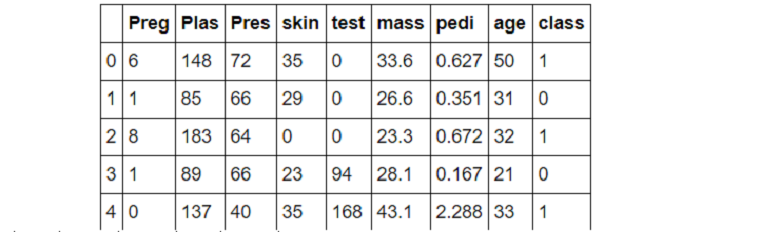``````iris_df.head(5)
``````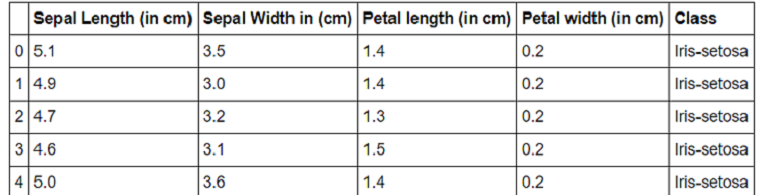### 3. Shape of a dataset

The shape of the dataset is basically a representation of total rows and columns present in the dataset. You can explore .shape() function present in the pandas' package here. In the Pima diabetic dataset, we have 768 rows and 9 columns, similarly, in the iris dataset, we have around 150 rows and 5 columns.

``````print(pima_df.shape)
print(iris_df.shape)
``````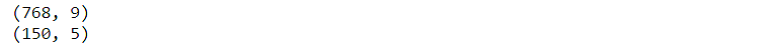### 4. Descriptive statistics of the data-sets

In pandas, describe() function is used to view central tendency, mean, median, standard deviation, percentile & many other things to give you the idea about the data.

``````pima_df.describe().transpose()

``````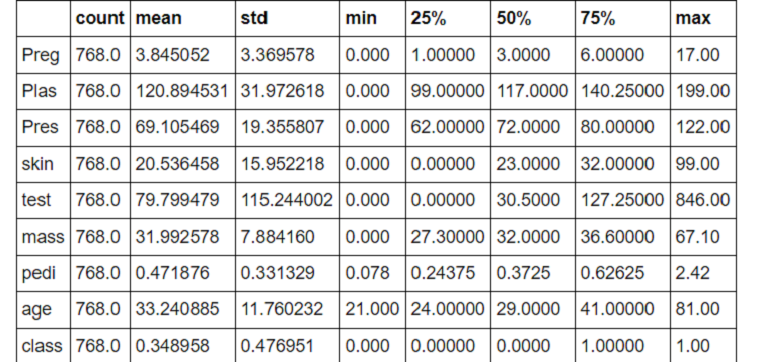``iris_df.describe().transpose()``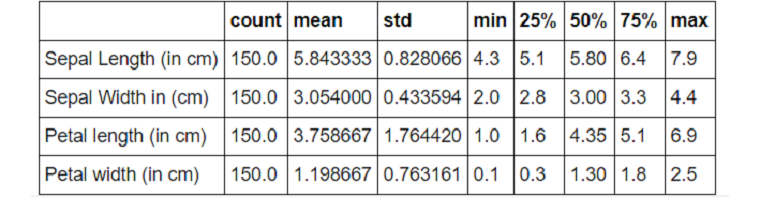(Must read: What is descriptive Analysis?)

### 5. Checking about the correlation between features in a dataset

There is a function in the panda's package which allows you to check about the correlation between features which is pd.DataFrame.corr(). It calculates the correlation between features pairwise excluding null values. I have used this function to compute the correlation between features in the Pima dataset which is shown in the below image.

``pima_df.corr()``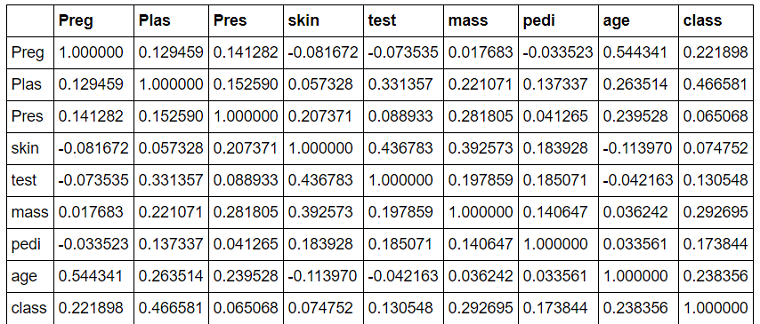(Must catch: What is Regression analysis?)

There is a function present in the pandas' package known as pd.dataFrame.info() which returns the data type of each column present in the dataset. Also, it tells you about null and not null values present. So, in our dataset, we have even int64 data types values and also float64 data type values.

``pima_df.info()``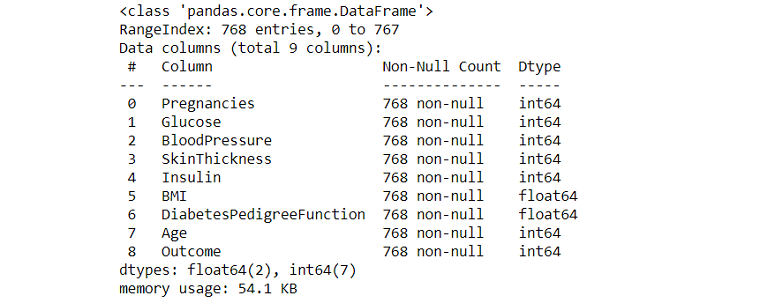(Related blog: Data types in Python)

### 7. Checking about missing values in the data

Missing values in the data can be checked by using isnull() function present in pandas documentation. It returns the boolean values that are true and false. If you want to calculate how many missing values are present in each column in the data set you can make use of the function isnull().sum(). This function returns the total number of missing values in each column.

In our case, in both the data sets we did not get any of the missing values in any of the columns.

``pima_df.isnull().sum()``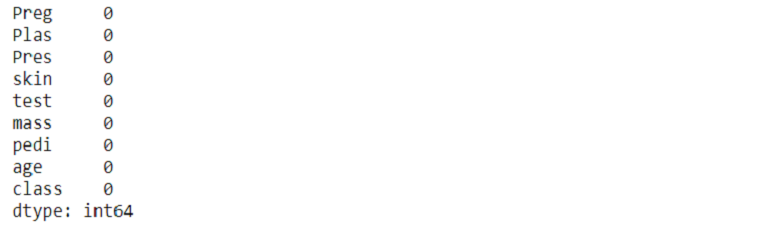### 7. If missing values are present then how to impute them?

For various scenarios, while dealing with data you will come across real-world data which will have missing values like nan values, -, blanks. The basic approach to deal with such a situation is to drop/ remove the entire row or column which contains missing values.

But dropping is not advisable because there will be a loss of data as well which can result in important parts of the data being removed. So, to deal with such things there are different methods used to impute the missing values.

There are two ways by which missing values can be imputed: the first is called univariate imputation and the other one is multivariate imputation.

• Univariate imputation is a type of imputation which imputes missing values considering only the non-missing values in that feature dimension. (e.g. impute.SimpleImputer).
• On the other hand, a multivariate imputer imputes the missing values considering all available features dimensions.(e.g. impute.IterativeImputer).

### 8. Encoding categorical features

Often it is seen that we do not have continuous values in our features. There are sometimes categorical values. And the system cannot understand such values so there is a need to convert them to continuous numerical values.

As seen in the below iris data frame we have classes as categorical features which are - ‘Iris-setosa’, ‘Iris-versicolor’, ‘Iris-virginica’.

#### How to encode them?

There are several different techniques that are used to encode categorical values which are stated below:

a) LabelEncoder() - It is a function present in the scikit- learn library of python which is used to convert categorical values in numerical values.

``````from sklearn.preprocessing import LabelEncoder,OneHotEncoder
LE = LabelEncoder()
OE= OneHotEncoder()
iris_df['Class'] = LE.fit_transform(iris_df['Class'])
iris_df['Class'].unique()``````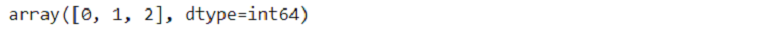Here we have imported LabelEncoder from sklearn.preprocessing followed by initialising of the object through which we will use the label encoder. We have made an object called “LE”. Then we have transformed our class column by using the LE.fit_transform function & printed the transformed class which is now [0,1,2]. It has given the values to Iris-setosa - 0, Iris-versicolor  - 1 , Iris-virginica - 2.

b) get_dummies() - Converts categorical features into dummy variables.

c) OneHotEncoder() - Array-like of integers or strings is the required input for this encoder. The features are encoded using a one-hot encoding scheme. The result is a binary column for each category and reverts a sparse matrix.

(Referred blog: What is Hierarchical Clustering in Machine Learning?)

### 9. Standardization of data

Standardization of data is a major important step that is required for machine learning algorithms to give good results. There are different scaling functions present in the preprocessing module of sci-kit learn. If data is not scaled and is passed to the algorithm the result might be wrong due to wrongly distributed data.

#### Why is it important to scale the data?

It is usually seen that we ignore checking the shape of the data distribution and change the data to be centred. That is done by removing the mean values of each column and then scaling it by dividing non-constant columns by their standard deviation.

Different functions are used by algorithms to learn to assume that all the desired features are centred as zero and also their variance is in the same structure. If any of the features have a higher proportion than all other features it may dominate the function for learning algorithm and does not allow learning from other features as required.

a) Scale:  present in the pre-processing module gives a fast and effective way to do this operation on a single array-like data:

``````X= np.array([[ 1, -1,  3],
[ 5,  0,  0],
[ 0,  2, -1]])
X_scaled = preprocessing.scale(X_train)
X_scaled
``````X_scaled has now unit variance and zero mean as you can see in the below image.

``````X_scaled.mean(axis=0)

array([0., 0., 0.])``````

b) The pre-processing module also has different other classes like StandardScaler that are used in scaling the data that is converting the mean to be zero and standard deviation to be united on training data which can be further used in test data as well. Such a class can also be used in building pipelines also.

The code implementation of the standard scaler is shown below.

`````` Y = np.array([[ 1., -1.,  2.],
[ 2.,  0.,  0.],
[ 0.,  1., -1.]])
Std = preprocessing.StandardScaler()
Y_scaled = Std.fit_transform(Y)
Y_scaled.mean(axis=0)

array([0., 0., 0.])``````

c) Scaling features to a range:  There are other methods also to scale data within a respective range that is a min value and max value. It mainly ranges between 0 and 1. You can use MinMaxScaler or MaxAbsScaler for scaling the data respectively.

(Must read: L2 and L1 Regularization in Machine Learning)

d) Scaling sparse data: Centering the scatter data would result in knock-down of sparsity structure of data thus it is not advisable to do. MinMaxScaler and MaxAbs scaler were introduced to scale the sparse data. Scalar often accepts both CSR (Compressed Sparse Rows) & also CSC (Compressed Sparse Columns).

If there is any other different sparse input then it is converted to Compressed Sparse Rows. To take care of the memory it is advisable to convert it in CSR and CSC representation.

e) Scaling data with the presence of outliers: If the data has outliers in it then scaling that sort of data using mean and variance is not a good approach. You can use robust_scale & Robust_Scaler as drop-in substitution.

### 10. Normalization of data

It is the process of scaling each sample to have a unit standard. These types of techniques are much more effective if you are computing the similarity between different pairs of samples or using a quadratic form like a dot product. This is the base of models used in text classifications. As discussing text classification, learn more about text mining and text mining techniques.

There is a function in the pre-processing module that is normalized which provides a good way to execute such operations on single array-like data by using L1 or L2 standards. Implementation of normalizing data using normalize is shown in the below image.

`````` X = [[ 2, -1.,  1],
[ 5.,  1,  0],
[ 0.,  1., -1]]
X_normalized = preprocessing.normalize(X, norm='l2')
X_normalized
``````Pre-processing module also has another class that is called a normalizer that executes similar operations using the transformer API. This class can also be used in the initial stage of the pipeline. Implementation of the normalizer is shown below in the image.

``````X = [[ 2, -1.,  1],
[ 5.,  1,  0],
[ 0.,  1., -1]]
norm= preprocessing.Normalizer()
norm.fit_transform(X)``````If you want to look for code implementation of EDA discussed above you can refer to the GitHub link here. It contains a jupyter file and both the datasets which are used.

## Conclusion

In this blog, I have tried to explain some operations which are done in exploratory data analysis to get a better understanding of the data. Techniques like missing values, standardization, normalization, shape, correlation between independent features also descriptive statistics of the data are discussed.

There can be various other things that can be done in EDA to get a better understanding that is dependent on what type of data we have. EDA in textual data or image data is entirely different which will be covered in different blogs dedicated to the image or textual data.

•#### 4mytempuse

Jul 11, 2020

I’m a Professional and experienced Data Analyst. I will do EDA(Exploratory Data Analysis) for businesses to take important decision. contact me here :- https://www.fiverr.com/biren_karena/do-data-analysis-visualization-and-generate-report

•